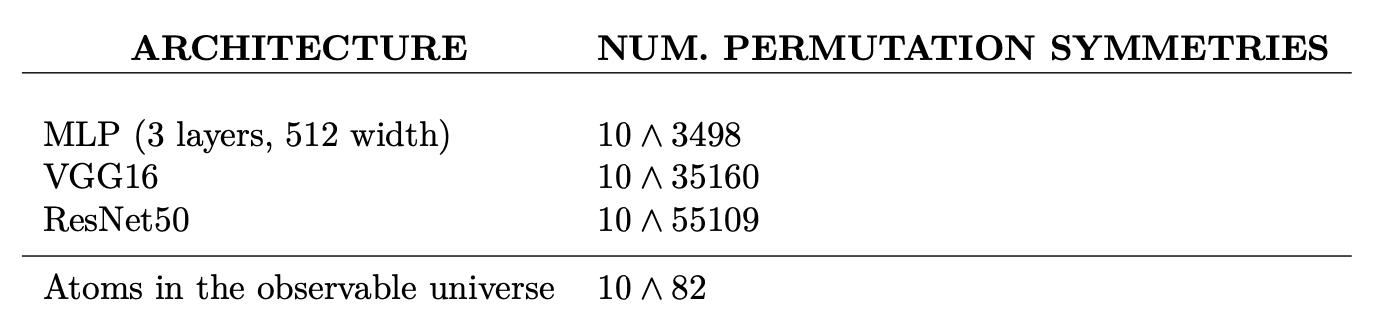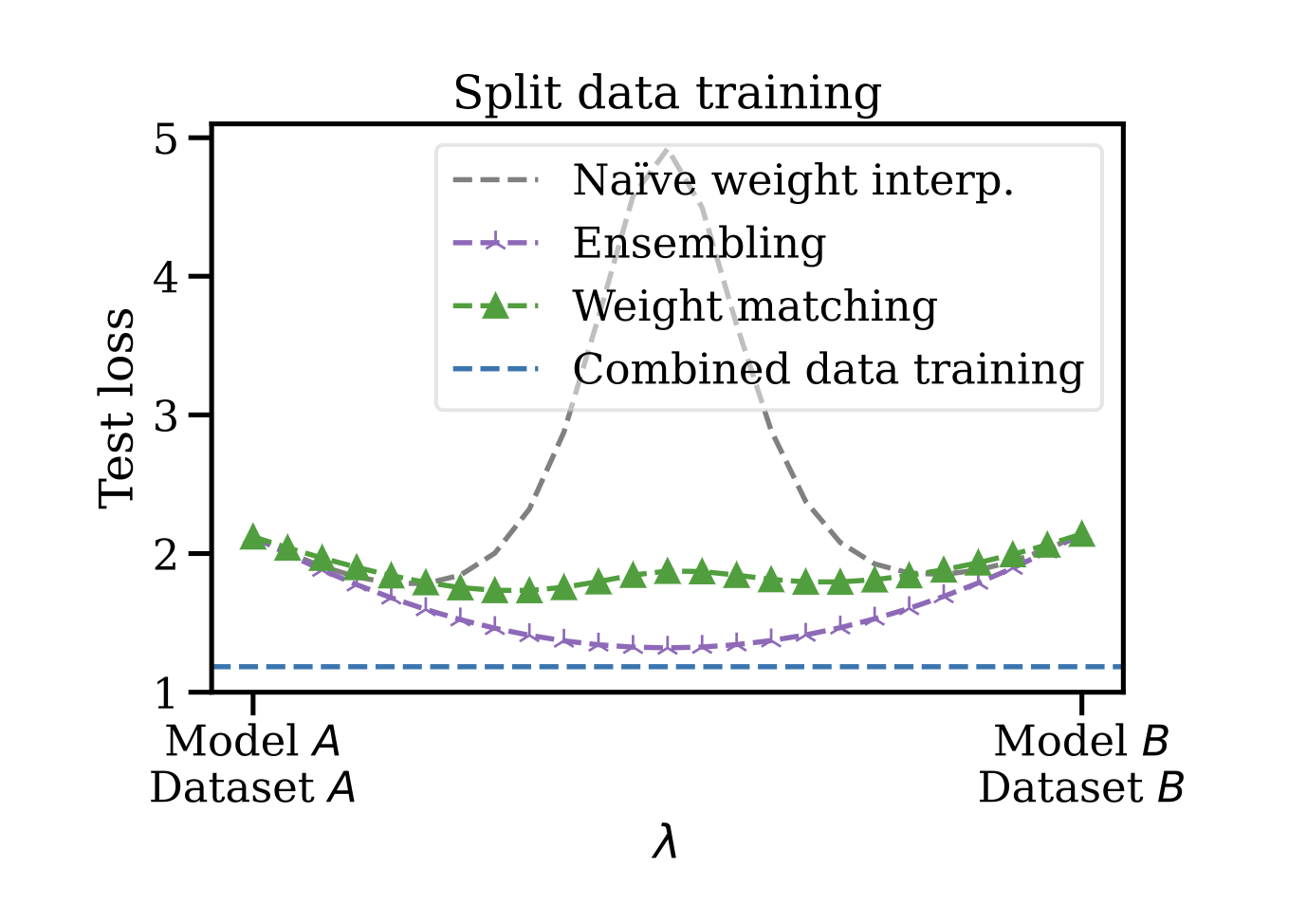# 21

See Arxiv and Twitter. From the abstract:

We argue that neural network loss landscapes contain (nearly) a single basin, after accounting for all possible permutation symmetries of hidden units. We introduce three algorithms to permute the units of one model to bring them into alignment with units of a reference model. This transformation produces a functionally equivalent set of weights that lie in an approximately convex basin near the ref- erence model. Experimentally, we demonstrate the single basin phenomenon across a variety of model architectures and datasets...

They point out that neural networks are highly symmetric, and that many permutations of the same weights can calculate the same function:

Brea et al. (2019) noted the permutation symmetries of hidden units in neural networks. Briefly: one can swap any two units of a hidden layer in a network and – assuming weights are adjusted accordingly – the functionality of the network will remain unchanged. Recently, Entezari et al. (2021) conjectured that these permutation symmetries may allow us to linearly connect points in weight space with no detriment to the loss.There's more than one way to shear a sheep, and a few more ways than that to arrange identical weights of a neural network such that you compute the same function.

Therefore, the difference between independent neural networks might not be the function they compute, but rather the permutation of their weights. In that case, it should be possible to find a mapping between the weights of two models that allows you to improve performance by interpolating between those weights. See here:

Conjecture 1 (Permutation invariance, informal (Entezari et al., 2021)). Most SGD solutions belong to a set whose elements can be permuted in such a way that there is no barrier (as in Definition 2.2) on the linear interpolation between any two permuted elements.

Definition 2.2 (Loss barrier (Frankle et al., 2020)). Given two points ΘA,ΘB such that L(ΘA) ≈ L(ΘB), the loss barrier is defined as:

They propose three techniques to find mappings between the neurons of different models that will allow interpolation to monotonically improve performance. These techniques are the technical core of the paper, outlined in Section 3. The first matches the activations of neurons on input data; the second matches the weights without examining activations; and the third uses a straight-through estimator. The latter two goals are shown to be very computationally expensive and, while their proposed solutions achieve success, this seems like one of the shortcomings of the current approach.

An experiment showed the success and limitations of their current technique's. They trained two ResNets on disjoint subsets of CIFAR-100, matched the weights between the models, permuted the weights of one of the models to mirror the other model, and performed linear interpolation. The technique performs much better than naive interpolation without weight matching, but not as well as ensembling the outputs of the independent models and worse still than training one model on the full dataset.Weight matching is better than naive interpolation, but not as good as ensembling or training on the full dataset.

Practical applications for this technique could include any opportunity to aggregate models trained on disjoint datasets. Data regulations and privacy concerns can limit opportunities to train one central model on all available data, but this kinds of technique can allow federated learning approaches of training separate models and merging them.

I'm more interested in what this tells us about the loss landscape of neural networks, and the potential for generalization. I won't speculate here, but consider the three questions provided as motivation at the top of the paper:

1. Why does SGD thrive in the optimization of high-dimensional non-convex deep learning loss land- scapes, despite being noticeably less robust in other non-convex optimization settings like policy learning (Ainsworth et al., 2021), trajectory optimization (Kelly, 2017), and recommender systems (Kang et al., 2016)?
2. Where are all the local minima? When linearly interpolating between initialization and final trained weights, why does the loss smoothly, monotonically decrease (Goodfellow & Vinyals, 2015; Frankle, 2020; Lucas et al., 2021; Vlaar & Frankle, 2021)?
3. How is it that two independently trained models with different random initializations and data batch orders inevitably achieve nearly identical performance? Furthermore, why do their training loss curves look identical?

New Comment